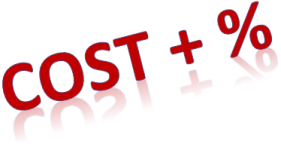# Cost-plus Pricing## Calculating Cost-plus pricing

To determine price using the cost-plus method:
Cost-plus price = (Total cost of production + mark-up) / Total output

For example if you are producing and selling bags and your cost to produce 200 bags was \$5,000 and you want to make 10% profit on each bag, you would determine your price as follows:

(\$5,000 + mark-up) / 200
(\$5,000 +(\$5,000*10%) / 200
(\$5,000 + \$500 ) / 200
= \$5500 / 200
= \$27.50

Alternately, you can also calculate as below:

(\$5,000 * 1.10) / 200
= \$5,500 / 200
= \$27.50

Therefore, in order to make a 10% profit on each bag you will need to price each at \$27.50.